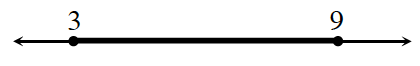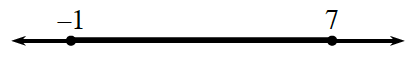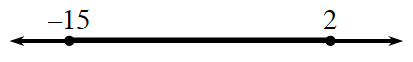### Home > GC > Chapter 7 > Lesson 7.3.2 > Problem7-113

7-113.

Each of these number lines shows a segment in bold. Find the midpoint of the segment in bold. (Note that the diagrams are not to scale.)

1.2.3.The midpoint is the mean, or average. What is the mean of each of these pairs of numbers?

$\frac{\left(3+9\right)}{2}=6$